Home / Physics / Electromotive Force (EMF)

# Electromotive Force (EMF)

## What is Electromotive Force (EMF)

Electromotive force, or emf, is the energy required to move a unit electric charge by an energy source such as a battery, cell, or generator. It is defined as the potential difference across the terminals where there is no current passing through it, i.e., an open circuit with one end positive and the other end negative.

In reality, the electromotive force is not a force but a measure of energy. The source converts one form of energy into electrical energy. For example, a battery converts chemical energy, and a generator converts mechanical energy.Electromotive Force EMF

The term electromotive force was coined by Italian physicist and chemist Alessandro Volta, who invented the electric battery in 1800.

## Electromotive Force (EMF) Equation

Suppose a circuit consists of a battery and a resistor. The electromotive force can be calculated using Kirchhoff’s Voltage Law. The following formula gives its value.

Symbol: ε, Greek letter epsilon

Formula:ε = IR + Ir

Where,

I: Current passing through the circuit

R: Resistance of the resistor

r: Internal resistance of the battery

Using Ohm’s law,

V = IR

Therefore,

ε = V + Ir

A voltmeter is used to measure the EMF.

SI Unit of Electromagnetic Force

The electromotive force is measured in Volt, which is its SI unit. A Volt is defined as Joule per Coulomb.

Volt = Joule/Coulomb

Dimension of Electromagnetic Force

The dimension of electromotive force is [M L2 T-3 I-1]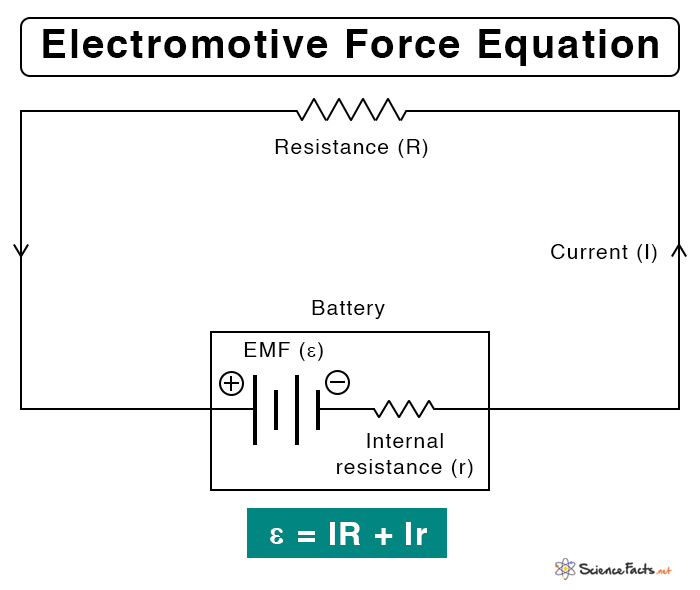Electromotive Force EMF Equation Formula

## Electromotive Force (EMF) vs. Voltage

As mentioned earlier, the electromotive force is the terminal potential difference when no current flows through it. The following table lists the differences between the emf and the potential difference or voltage.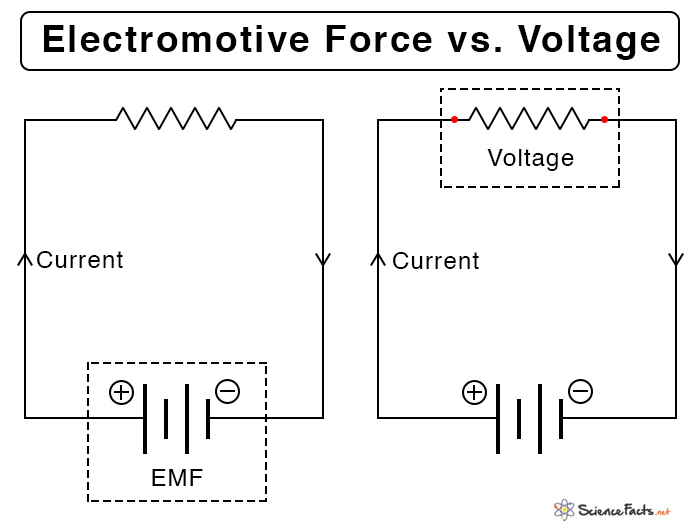Electromotive Force EMF vs Voltage

Article was last reviewed on Monday, April 5, 2021

### Related articlesNuclear Force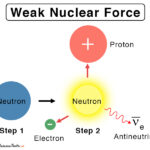Weak Nuclear Force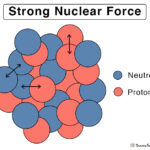Strong Nuclear Force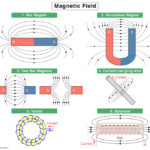Magnetic Field